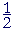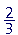S k i l l
i n
A R I T H M E T I C

Lesson 22

# EQUIVALENT FRACTIONS

 1. What are equivalent fractions? Equivalent fractions have different names but the same value; they are at the same place on the number line. They have the same relationship to 1.

Here is an elementary example:

 12 = 24 .12 is 1 of 2 equal parts of 1. 24 is 2 of 4 equal parts.  Each

fraction is one half of 1.

 2. How can we know when fractions are equivalent?Both the numerator and denominator of one fraction have been multiplied or divided by the same number. Those products or quotients are the numerator and denominator of the equivalent fraction.
 23 and 1015 are equivalent fractions.

Every fraction has a ratio to 1. Equivlent fractions will have the same ratio to 1.

Since the numerator and denominator are natural numbers, they will have all the properties of ratios, specifically the theorem of the same multiple.

 Example 1.   Name three fractions that are equivalent to 56 .

 1012 1518 5060

To create them, we multiplied both 5 and 6 by the same number.  First by 2, then by 3, then by 10.

 3. How do we convert a fraction to an equivalent fraction with a larger denominator?Multiply the original denominator so that it equals the larger denominator. Multiply the numerator by the same number.
 34 = 6 × 36 × 4 = 1824 .

(Compare Lesson 20, Problem 2c.)

Example 2.    Write the missing numerator:

 67 = ? 28

Answer.   To make 7 into 28, we have to multiply it by 4.  Therefore, we must also multiply 6 by 4:

 67 = 244 × 6284 × 7

In practice, to find the multiplier, mentally divide the original denominator into the new denominator, and then multiply the numerator by that quotient.  That is, say:

"7 goes into 28 four times. Four times 6 is 24."

Compare Lesson 18, Example 5.  Every property of ratios applies to fractions.

Example 3.   Write the missing numerator:

 58 = ? 48

Answer.   "8 goes into 48 six times.  Six times 5 is 30."

 58 = 3048 .

In actual problems, we convert two (or more) fractions so that they have equal denominators.  When we do that, it is easy to compare them. Moreover, equal denominators are necessary in order to add or subtract fractions.  For we can only add or subtract quantities that have the same name, that is, that are units of the same kind; and it is the denominator of a fraction that names the unit. (Lesson 21.)

Now, since 15, for example, is a multiple of 5, we say that 5 is a divisor of 15.

5 is also a divisor of 20.  5 is a common divisor of 15 and 20.

(15 and 22 have no common divisors, except 1, which is a divisor of every number.)

4.   What denominator should we choose
when the denominators of two fractions have no common divisors,
 23 and 58
and we want to convert them to equivalent fractions with equal denominators?

Choose the product of the denominators.

Example 4.   Convertandto equivalent fractions with equal denominators.

Answer.   The denominators 3 and 8 have no common divisors (except 1).  Therefore, as a common denominator, choose 24.

 23 = 1624 . 58 = 1524 .

To convertwe said, "3 goes into 24 eight times. Eight tmes 2 is 16."

To convertwe said, "8 goes into 24 three times. Three times 5 is 15."

Once we convert to a common denominator, we could then know thatis greater than. Because when fractions have equal denominators, then the larger the numerator, the larger the fraction. (Lesson 20, Question 11.)

Also, we could now add those fractions:

 23 + 58 = 1624 + 1524 = 3124 .

We can choose the product of denominators even when the denominators have a common divisor. But their product will not then be their lowest common multiple (Lesson 23). The student should prefer the lowest common multiple because smaller numbers make for simpler calculations.

Same ratio

When fractions are equivalent, their numerators and denominators are in the same ratio.  That in fact is the best definition of equivalent fractions.

 12 = 24 .

1 is half of 2.  2 is half of 4.  In fact, any fraction where the numerator is half of the denominator will be equivalent to.

 12 = 24 = 36 = 5 10 = 8 16 .

1 is half of 2.  2 is half of 4.  3 is half of 6.  5 is half of 10.  And so on.  They are all at the same place on the number line.Example 5.andare equivalent because each numerator is a third of its denominator.

Example 6.   Write the missing numerator:

 7 28 = ? 16

Answer.  7 is a quarter of 28.  And a quarter of 16 is 4.

 7 28 = 4  16 .

7 is to 28  as  4 is to 16.

 7 28 and 4 16 are equivalent.

How to simplify, or reduce, a fraction

The numerator and denominator of a fraction are called its terms. To simplify or reduce a fraction means to make the terms smaller numbers.  To do that, we divide both terms by a common divisor.

 Example 7. 2436 = 24 ÷ 436 ÷ 4 = 69 = 6 ÷ 39 ÷ 3 = 23 .
 2436 , 69 ,  and 23 are equivalent fractions.

Of those threehas the as the lowest terms. We cannot divide any further.

We like to express a fraction with its lowest terms because it gives a better sense of its value, and it makes for simpler calculations.

 5. How do we reduce a fraction to its lowest terms? Keep dividing both terms by a common divisor. Or, take the same part of both terms.

 Example 8.   Reduce to lowest terms: 15 21 .

Answer.  15 and 21 have a common divisor, 3.

 1521 = 5"3 goes into 15 five times."7"3 goes into 21 seven times."

Or, take a third of both 15 and 21.

"A third of 15 is 5."

"A third of 21 is 7."

 Example 9.   Reduce 200 1200 .

Answer.  When the terms have the same number of 0's, we may ignore them.

 2001200 = 16

Effectively, we have divided 200 and 1200 by 100. (Lesson 2, Question 10.)

 Example 10.   Write as a mixed number: 20 8 .

Solution.  Divide 20 by 8.  "8 goes into 20 two (2) times (16) with 4 left over."

 20 8 =  2 48 =  2 12 .
 48 is equivalent to 12 .

Or, we could reduce first.  20 and 8 have a common divisor 4:

 20  8 = 5"4 goes into 20 five times."2"4 goes into 8 two times." = 2½.

Notice that we are free to interpret the same symbol

 20 8
 in various ways.  It is the fraction 20 8 .  It is 20 divided by 8.

And it indicates "the ratio of 20 to 8."

 Example 11.   Reduce 55 .

Any fraction in which the numerator and denominator are equal, is equal to 1.

 SummaryAt this point, please "turn" the page and do some Problems.

or

Continue on to the next Section.